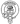# The correct way to shuffle an array in JavaScript

August 11, 2020

In a recent project I was working on I had a list of objects in an array and wanted to be able to display them in a random order. While browsing around for a solution I came across quite a few implementations that while short and simple to comprehend were either inefficient or strongly biased. Take this simple example:

``````const numbers = [1, 2, 3, 4, 5, 6, 7]

const shuffledNumbers = numbers.sort(() => 0.5 - Math.random())

console.log(shuffledNumbers)

// [3, 2, 4, 7, 6, 1, 5]``````

At first glance all seems to be well, our array appears to be a random order but the problem is it’s not really random at all! Every permutation is not equally likely. Luckily for us we aren’t the first people to try to shuffle an array of values and there’s a well known and verified solution, the Fisher-Yates Algorithm. Originally described in 1938 and modernized for computer use by Richard Drustenfield in 1964, it can explained like this:

``````-- To shuffle an array a of n elements (indices 0..n-1):
for i from n−1 downto 1 do
j ← random integer such that 0 ≤ j ≤ i
exchange a[j] and a[i]``````

but how do we then implement this in JavaScript? Well it’s actually remarkably simple and can be done all within one for loop.

``````const shuffle = arr => {
let copy = arr.slice(0)
for (var i = copy.length - 1; i > 0; i--) {
let j = Math.floor(Math.random() * (i + 1))
let tmp = copy[i]
copy[i] = copy[j]
copy[j] = tmp
}

return copy
}

console.log(shuffle([1, 2, 3, 4, 5, 6, 7]))

// [3, 5, 1, 2, 4, 7, 6]``````

This can be further improved as ES6 allows us to assign to two variables at the same time, making switching the values of `array[i]` and `array[j]` more simpler and succinct.

``````const shuffle = arr => {
let copy = arr.slice(0)
for (let i = copy.length - 1; i > 0; i--) {
const j = Math.floor(Math.random() * (i + 1))
;[copy[i], copy[j]] = [copy[j], copy[i]]
}
return copy
}

console.log(shuffle([1, 2, 3, 4, 5, 6, 7]))

// [6, 2, 5, 1, 4, 7, 3]``````Written by Fraser Hamilton a full stack developer based out of Edinburgh, Scotland.